StatLect

# Stationary sequence

A sequence of random variables is said to be stationary if the joint distribution of a group of adjacent terms of the sequence does not depend on the position of the group within the sequence.## Synonyms

A stationary sequence is also called:

• strictly stationary sequence;

• strongly stationary sequence;

• stationary process;

• strictly stationary process;

• strongly stationary process.

## Definition

The following is a formal definition.

Definition A sequence of random variablesis said to be stationary if and only if the two random vectors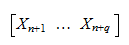and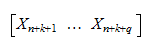have the same joint distribution for any,and.

So, for example, the two vectors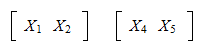must have the same joint distribution, and so must the vectors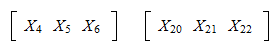## Identical distributions

By settingin the definition above, we can see that if a sequence is strictly stationary, then all the random variables in the sequence have the same distribution.

In other words,andhave the same distribution for anyand. They also have the same expected value and the same variance, provided that these moments exist.

## IID sequence

The simplest example of a strictly stationary process is an IID sequence, that is, a sequence whose terms are mutually independent and have the same distribution.

Proof

Denote bya (joint) distribution function. Then,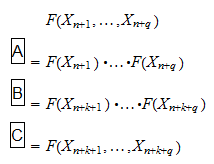where: in stepsandwe have used the fact that the joint distribution function of a set of mutually independent variables is equal to the product of their marginal distribution functions; in stepwe have used the fact that all the terms of the sequence have the same distribution.

## Sequences of identically distributed variables

A sequence of identically distributed variables can be not stationary.

Let us make an example.

Suppose thathas a standard normal distribution (zero mean and unit variance).

DefineIn other words, each of the first ten terms of the sequence is equal to the previous term changed in sign.

Then, there is no change in sign for the subsequent terms: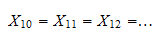Switching the sign of a zero-mean normal variable does not change its distribution. Therefore, all the terms of the sequence have the same distribution.

However,is not a strictly stationary process because, for example, the vectorand the vectordo not have the same joint distribution.

## Use in statistics

The assumption of strict stationarity is used to prove several important results in statistics, for example:

## Relation between strong and weak stationarity

A related concept is that of weak (or covariance) stationarity.

A process is said to be weakly stationary if and only if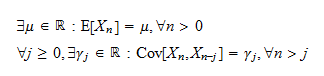In this definition, both the meanand the autocovariancesof the process are required to be finite.

If a strictly stationary process satisfies these finiteness conditions, then it is also weakly stationary.

Proof

We have already said that the variables of a strictly stationary process all have the same distribution. Therefore,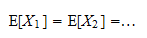or, if we consider that the mean is assumed to be finite, there existssuch thatfor any. Moreover, the vectorhas the same joint distribution for anyand any. Therefore, if the covariance betweenandis finite, we have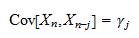for anyand.

Clearly, a weakly stationary process needs not be strongly stationary.

A simple counterexample is a sequence of independent random variables all having a t distribution with the same mean, the same variance but different degrees of freedom parameters. Such a sequence is weakly, but not strongly stationary.

## Multivariate generalization

The concept extends in a straightforward manner to sequences of random vectors.

The definition remains exactly the same we have given above, except for the fact that the components ofandare themselves vectors.

## More details

To learn more about the concepts explained here and other related concepts, you can read the lectures on:

## Keep reading the glossary

Previous entry: Standard deviation

Next entry: Statistical model